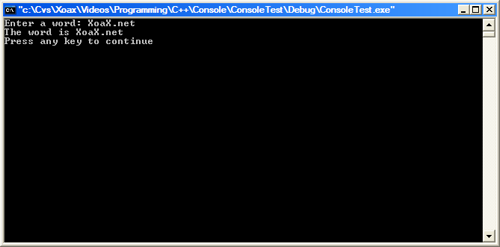• Programming
• Internet

# Using STL Strings

Example 1

```// Inputting and outputting a simple string
#include <iostream>
#include <string>

int main() {
using namespace std;

// Enter text into a string
string qWord;
cout << "Enter a word: ";
cin >> qWord;
cout << "The word is " << qWord << endl;

return 0;
}
```Example 2

```// Converting from string to double and double to string
#include <iostream>
#include <string>
#include <sstream>

int main() {
using namespace std;

// Conversion from string to double
stringstream qStringToDouble;
string qString("3.14159");
qStringToDouble << qString;
double dConvertedDouble;
qStringToDouble >> dConvertedDouble;
// Output the double result
cout << dConvertedDouble << endl;

// Conversion from double to string
stringstream qDoubleToString;
double dDouble = 2.71828;
qDoubleToString << dDouble;
string qConvertedString(qDoubleToString.str());
// Output the string
cout << qConvertedString << endl;

return 0;
}
```Example 3

```// In this example a word is entered into a string.
// A mirrored version of the word is created using iterators
// Then a check is made as whether the word is a palindrome.
#include <iostream>
#include <string>

int main() {
using namespace std;

// Enter text into a string
string qWord;
cout << "Enter a word: ";
cin >> qWord;

// Use reverse iterators to mirror the word
string qReversed(qWord.rbegin(), qWord.rend());
cout << "Backwards, it is: " << qReversed << endl;

// Use == to check whether it is a palindrome
if (qWord == qReversed) {
cout << qWord << " is a palindrome" << endl;
} else {
cout << qWord << " is not a palindrome" << endl;
}

return 0;
}
```Example 4

```// Example of code sorting several strings in alphabetical order
#include <iostream>
#include <string>
#include <vector>
#include <algorithm>

int main() {
using namespace std;

string qName1("XoaX.net");
string qName3("C++");
string qName2("Algorithms");
string qName4("Graphics");

vector<string> qNames;
qNames.push_back(qName1);
qNames.push_back(qName2);
qNames.push_back(qName3);
qNames.push_back(qName4);

cout << "Unsorted list:" << endl;
for (unsigned int uiIndex = 0; uiIndex < 4; ++uiIndex) {
cout << qNames[uiIndex] << endl;
}

sort(qNames.begin(), qNames.end());

cout << endl << "Sorted list:" << endl;
for (unsigned int uiIndex = 0; uiIndex < 4; ++uiIndex) {
cout << qNames[uiIndex] << endl;
}

return 0;
}
```Example 5

```// Examples of finding strings within strings
#include <iostream>
#include <string>

int main() {
using namespace std;

// A famous quote from Alexander Pope's "An Essay on Criticism"
string qPopeQuote("A little learning is a dangerous thing.");
// Find the position (3) of the first "i"
cout << (unsigned int)qPopeQuote.find('i') << endl;

// Another famous quote from Alexander Pope's "An Essay on Criticism"
string qPopeQuote2("To err is human; to forgive, divine.");
string qLetters("dfimh");
// Find the position (7) of the first of the letters "dfimh"
cout << (unsigned int)qPopeQuote2.find_first_of(qLetters) << endl;

// A couplet from Alexander Pope's "An Essay on Man" in two lines
string qPopeLine1("If white and black blend, soften and unite");
string qPopeLine2("a thousand ways, is there no black or white?");
// Put the two lines together in a single string
string qPopeQuote3(qPopeLine1 + qPopeLine2);
string qBlack("black");
// Find the position (13) from the front of the word "black"
cout << (unsigned int)qPopeQuote3.find(qBlack) << endl;
// Find the position (71) from the back for the word "black"
cout << (unsigned int)qPopeQuote3.rfind(qBlack) << endl;

return 0;
}
```Example 6

```// An example of extracting and erasing a substring
#include <iostream>
#include <string>

int main() {
using namespace std;

// A quote from Ayn Rand's "Atlas Shrugged"
string qRand1("There are two sides to every issue: one side is right\n");
string qRand2("and the other is wrong, but the middle is always evil.");
// Append the second half of the quote to the first.
qRand1.append(qRand2);
cout << qRand1 << endl;

// Extract the substring: "one side is right"
string qSubstring = qRand1.substr(36, 17);
cout << qSubstring << endl;

// Remove the middle string:
// ": one side is right\nand the other is wrong"
// This leaves the sentence as.
// "There are two sides to every issue, but the middle is always evil."
qRand1.erase(34, 42);
cout << qRand1 << endl;

return 0;
}
```